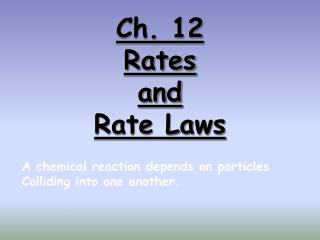Download PresentationCh. 12 Rates and Rate Laws

# Ch. 12 Rates and Rate Laws - PowerPoint PPT PresentationDownload Presentation## Ch. 12 Rates and Rate Laws

- - - - - - - - - - - - - - - - - - - - - - - - - - - E N D - - - - - - - - - - - - - - - - - - - - - - - - - - -
##### Presentation Transcript

1. Ch. 12RatesandRate Laws A chemical reaction depends on particles Colliding into one another.

2. Reaction Rate The change in concentration of a reactant or product per unit of time

3. Reaction Rates: 2NO2(g)  2NO(g) + O2(g) 1. Can measure disappearance of reactants 2. Can measure appearance of products 3. Are proportional stoichiometrically

4. Reaction Rates: 2NO2(g)  2NO(g) + O2(g) 4. Are equal to the slope tangent to that point 5. Change as the reaction proceeds, if the rate is dependent upon concentration [NO2] t

5. Rate Laws Differential rate lawsexpress (reveal) the relationship between the concentration of reactants and the rate of the reaction. The differential rate law is usually just called “the rate law.” Integrated rate lawsexpress (reveal) the relationship between concentration of reactants and time

6. What does a Rate Law look like? Rate = k[A] [B] k, is the rate law constant Exponent is the order of each reactant Adding exponents gives the overall rate order

7. Determining Order withConcentration vs. Time data (the Integrated Rate Law) Zero Order: has a constant rate First Order: Half-life is constant, doubling conc. will double reaction rate Second Order: Doubling the concentration, will quadruple the rxn rate

8. Writing a (differential) Rate Law Problem- Write the rate law, determine the value of the rate constant, k, and the overall order for the following reaction: 2 NO(g) + Cl2(g)  2 NOCl(g)

9. Writing a Rate Law Part 1– Determine the values for the exponents in the rate law: R = k[NO]x[Cl2]y In experiment 1 and 2, [Cl2] is constant while [NO] doubles. The rate quadruples, so the reaction is second order with respect to [NO]  R = k[NO]2[Cl2]y

10. Writing a Rate Law Part 1– Determine the values for the exponents in the rate law: R = k[NO]2[Cl2]y In experiment 2 and 4, [NO] is constant while [Cl2] doubles. The rate doubles, so the reaction is first order with respect to [Cl2]  R = k[NO]2[Cl2]

11. Writing a Rate Law Part 2– Determine the value for k, the rate constant, by using any set of experimental data: R = k[NO]2[Cl2]

12. Writing a Rate Law Part 3– Determine the overall order for the reaction. R = k[NO]2[Cl2] = 3 2 + 1  The reaction is 3rd order Overall order is the sum of the exponents, or orders, of the reactants

13. Classwork • P.581 #27-28

14. Graphs of Orders Value of K is given by the slope of the line. • P.553 First order: time vsln [A] • Linear with a negative slope • P.558 Second order: time vs 1/[A] • Linear with a positive slope • P.559 Zero order: time vs [A] • Linear with a negative slope

15. Solving an Integrated Rate Law Problem: Find the integrated rate law and the value for the rate constant, k A graphing calculator with linear regression analysis greatly simplifies this process!!

16. Time vs. [H2O2] Regression results: y = ax + b a = -2.64 x 10-4 b = 0.841 r2 = 0.8891 r = -0.9429

17. Time vs. ln[H2O2] Regression results: y = ax + b a = -8.35 x 10-4 b = -.005 r2 = 0.99978 r = -0.9999

18. Time vs. 1/[H2O2] Regression results: y = ax + b a = 0.00460 b = -0.847 r2 = 0.8723 r = 0.9340

19. And the winner is… Time vs. ln[H2O2] 1. As a result, the reaction is 1st order 2. The (differential) rate law is: 3. The integrated rate law is: 4. But…what is the rate constant, k ?

20. Finding the Rate Constant, k Method #1: Calculate the slope from the Time vs. ln[H2O2] table. Now remember:  k = -slope k = 8.32 x 10-4s-1

21. Finding the Rate Constant, k Method #2: Obtain k from the linear regresssion analysis. Regression results: y = ax + b a = -8.35 x 10-4 b = -.005 r2 = 0.99978 r = -0.9999 Now remember:  k = -slope k = 8.35 x 10-4s-1

22. Rate Laws Summary

23. Classwork • P.582 #35, 39